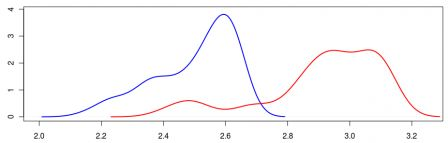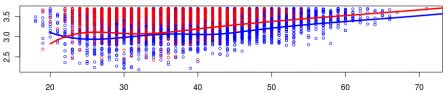Playing with quantiles, part 2

March 8, 2011
By

(This article was first published on Freakonometrics - Tag - R-english, and kindly contributed to R-bloggers)It is common to look at best
time at the Marathon. Or perhaps the distribution of the top100, as
done by John Myles White on his blog here
(data can be found there), as
the graph below, with the density of the time for the first 100 men (in blue) and the first 100 women
(in red).Hence, men and women are different and men run faster than women. So
what…?
A lot of people run those marathon. Almost 40,000 ran (and finished) the
one in New York City. Instead of 100, shouldn’t we keep more runners, e.g
the top 5% ?
The other point is that it might be interesting to correct (or control)
with the age. If men and women running the marathon do not have the
same age, it might be an explanation for that difference.
Unfortunately, to run a quantile regression properly I need the entire
dataset, with 40,000 runners. And on the website of the NY Marathon (here) it is
possible to extract some information, but not the entire dataset (and
my standard R robots did not work here). So I managed to extract the
“worst” 10% runners in 2008, and run a quantile regression to get the
95% quantile regression curve, using the idea mentioned here.I got the 95% quantile curve for men (in blue) and women (in red), and similarly for top runner, namely
the 5% quantile curve.On those graphs, if we exclude runners under 22, quantile curves for men
are usually below the ones for women. Which confirms the first order
dominance mentioned by John,
For those willing to play, here is the code (it is dirty since my
dataset was dirty)

`dataend=read.table("/home/arthur/NY2008-top.csv",header=FALSE,sep="t")nrow(dataend)I=dataend[,5]I[is.na(dataend[,5])]=dataend[is.na(dataend[,5]),6]q=which(substr(as.character(I),1,1)%in%c("M","F"))I=I[q]age=as.numeric(substr(as.character(I),2,3))sex=substr(as.character(I),1,1)=="M"tp=as.character(dataend[,45])i=which(tp=="");tp[i]=as.character(dataend[i,44])i=which(tp=="");tp[i]=as.character(dataend[i,43])i=which(tp=="");tp[i]=as.character(dataend[i,42])tp=tp[q]temps=((as.numeric(substr(tp,1,1))+as.numeric(substr(tp,3,4))/60)*26.21875)/60propend=length(tp)/n2008Menbase=data.frame(age,temps)base=base[sex==TRUE,]reg=rq(temps~bs(age,7),data=base,tau=.05/propend)u=seq(20,80)bu=data.frame(age=u)y2008m=predict(reg,newdata=bu)propend=length(tp)/n2008Womenbase=data.frame(age,temps)base=base[sex==FALSE,]reg=rq(temps~bs(age,5),data=base,tau=.05/propend)u=seq(20,80)bu=data.frame(age=u)y2008w=predict(reg,newdata=bu)`

But looking at archives (here 1983 compared with 2008) I noticed something
strange. First, if we compare number of runners (here starters),

 Men Women 1983 12,838 2,553 2008 25,669 13,163
it looks like more and more women run
the marathon nowadays. So this might have an impact on the results,On the graph above, the have a strong
line for 2008 and a thin one for 1983, and again men in blue and women in red. It looks like fast runners run as fast as before, but since there are more and more runners, the quantiles are going up, And by
that time, women who ran the marathon were performing extremely well
compared with men.
Anyway, this is now really a statistical analysis, but more a “how to say something when you cannot
access a database entirely
“. But I still hope someday I will…

R-bloggers.com offers daily e-mail updates about R news and tutorials on topics such as: Data science, Big Data, R jobs, visualization (ggplot2, Boxplots, maps, animation), programming (RStudio, Sweave, LaTeX, SQL, Eclipse, git, hadoop, Web Scraping) statistics (regression, PCA, time series, trading) and more...

Tags: , , , ,